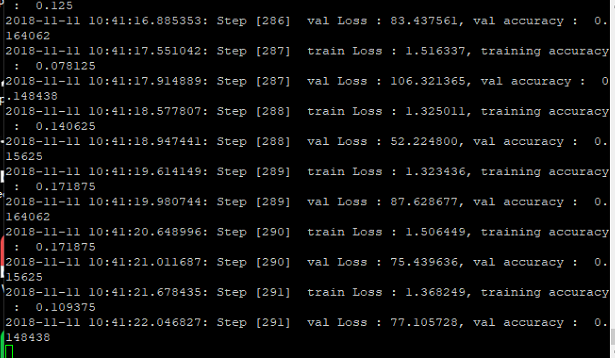`````` # coding=utf-8
import tensorflow as tf
import numpy as np
import pdb
import os
from datetime import datetime
import slim.inception_model as inception_v3
from create_tf_record import *
import tensorflow.contrib.slim as slim

labels_nums = 7  # 类别个数
batch_size = 64  #
resize_height = 299  # 指定SSS存储图片高度
resize_width = 299  # 指定存储图片宽度
depths = 3
data_shape = [batch_size, resize_height, resize_width, depths]

# 定义input_images为图片数据
input_images = tf.placeholder(dtype=tf.float32, shape=[None, resize_height, resize_width, depths], name='input')
# 定义input_labels为labels数据
# input_labels = tf.placeholder(dtype=tf.int32, shape=[None], name='label')
input_labels = tf.placeholder(dtype=tf.int32, shape=[None, labels_nums], name='label')

# 定义dropout的概率
keep_prob = tf.placeholder(tf.float32, name='keep_prob')
is_training = tf.placeholder(tf.bool, name='is_training')

#config = tf.ConfigProto()
#config = tf.ConfigProto()
#config.gpu_options.allow_growth = True
#tf.Session(config = config)
#tf.Session(config=tf.ConfigProto(allow_growth=True))

def net_evaluation(sess, loss, accuracy, val_images_batch, val_labels_batch, val_nums):
val_max_steps = int(val_nums / batch_size)
val_losses = []
val_accs = []
for _ in range(val_max_steps):
val_x, val_y = sess.run([val_images_batch, val_labels_batch])
# print('labels:',val_y)
# val_loss = sess.run(loss, feed_dict={x: val_x, y: val_y, keep_prob: 1.0})
# val_acc = sess.run(accuracy,feed_dict={x: val_x, y: val_y, keep_prob: 1.0})
val_loss, val_acc = sess.run([loss, accuracy],
feed_dict={input_images: val_x, input_labels: val_y, keep_prob: 1.0,
is_training: False})
val_losses.append(val_loss)
val_accs.append(val_acc)
mean_loss = np.array(val_losses, dtype=np.float32).mean()
mean_acc = np.array(val_accs, dtype=np.float32).mean()
return mean_loss, mean_acc

def step_train(train_op, loss, accuracy,
train_images_batch, train_labels_batch, train_nums, train_log_step,
val_images_batch, val_labels_batch, val_nums, val_log_step,
snapshot_prefix, snapshot):
'''
循环迭代训练过程
:param train_op: 训练op
:param loss:     loss函数
:param accuracy: 准确率函数
:param train_images_batch: 训练images数据
:param train_labels_batch: 训练labels数据
:param train_nums:         总训练数据
:param train_log_step:   训练log显示间隔
:param val_images_batch: 验证images数据
:param val_labels_batch: 验证labels数据
:param val_nums:         总验证数据
:param val_log_step:     验证log显示间隔
:param snapshot_prefix: 模型保存的路径
:param snapshot:        模型保存间隔
:return: None
'''
# 初始化
#init = tf.global_variables_initializer()
saver = tf.train.Saver()
max_acc = 0.0
#ckpt = tf.train.get_checkpoint_state('D:/can_test/inception v3/')
#saver = tf.train.import_meta_graph(ckpt.model_checkpoint_path + '.meta')
#tf.reset_default_graph()
with tf.Session() as sess:
#sess.run(tf.global_variables_initializer())#恢复训练用
#saver = tf.train.import_meta_graph('D://can_test/inception v3/best_models_2_0.7500.ckpt.meta')#恢复训练
#saver.restore(sess, tf.train.latest_checkpoint('D://can_test/inception v3/'))#恢复训练
sess.run(tf.global_variables_initializer())
sess.run(tf.local_variables_initializer())
coord = tf.train.Coordinator()
for i in range(max_steps + 1):
batch_input_images, batch_input_labels = sess.run([train_images_batch, train_labels_batch])
_, train_loss = sess.run([train_op, loss], feed_dict={input_images: batch_input_images,
input_labels: batch_input_labels,
keep_prob: 0.5, is_training: True})
# train测试(这里仅测试训练集的一个batch)
if i % train_log_step == 0:
train_acc = sess.run(accuracy, feed_dict={input_images: batch_input_images,
input_labels: batch_input_labels,
keep_prob: 1.0, is_training: False})
print(
"%s: Step [%d]  train Loss : %f, training accuracy :  %g" % (
datetime.now(), i, train_loss, train_acc)
)
# val测试(测试全部val数据)
if i % val_log_step == 0:
mean_loss, mean_acc = net_evaluation(sess, loss, accuracy, val_images_batch, val_labels_batch, val_nums)
print(
"%s: Step [%d]  val Loss : %f, val accuracy :  %g" % (datetime.now(), i, mean_loss, mean_acc)
)
# 模型保存:每迭代snapshot次或者最后一次保存模型
if  i == max_steps:
print('-----save:{}-{}'.format(snapshot_prefix, i))
saver.save(sess, snapshot_prefix, global_step=i)
# 保存val准确率最高的模型
if mean_acc > max_acc and mean_acc > 0.90:
max_acc = mean_acc
path = os.path.dirname(snapshot_prefix)
best_models = os.path.join(path, 'best_models_{}_{:.4f}.ckpt'.format(i, max_acc))
print('------save:{}'.format(best_models))
saver.save(sess, best_models)

coord.request_stop()

def train(train_record_file,
train_log_step,
train_param,
val_record_file,
val_log_step,
labels_nums,
data_shape,
snapshot,
snapshot_prefix):
'''
:param train_record_file: 训练的tfrecord文件
:param train_log_step: 显示训练过程log信息间隔
:param train_param: train参数
:param val_record_file: 验证的tfrecord文件
:param val_log_step: 显示验证过程log信息间隔
:param val_param: val参数
:param labels_nums: labels数
:param data_shape: 输入数据shape
:param snapshot: 保存模型间隔
:param snapshot_prefix: 保存模型文件的前缀名
:return:
'''
[base_lr, max_steps] = train_param
[batch_size, resize_height, resize_width, depths] = data_shape

# 获得训练和测试的样本数
train_nums = get_example_nums(train_record_file)
val_nums = get_example_nums(val_record_file)
print('train nums:%d,val nums:%d' % (train_nums, val_nums))

# 从record中读取图片和labels数据
# train数据,训练数据一般要求打乱顺序shuffle=True
train_images, train_labels = read_records(train_record_file, resize_height, resize_width, type='normalization')
train_images_batch, train_labels_batch = get_batch_images(train_images, train_labels,
batch_size=batch_size, labels_nums=labels_nums,
one_hot=True, shuffle=True)
# val数据,验证数据可以不需要打乱数据
val_images, val_labels = read_records(val_record_file, resize_height, resize_width, type='normalization')
val_images_batch, val_labels_batch = get_batch_images(val_images, val_labels,
batch_size=batch_size, labels_nums=labels_nums,
one_hot=True, shuffle=False)

# Define the model:
with slim.arg_scope(inception_v3.inception_v3_arg_scope()):
out, end_points = inception_v3.inception_v3(inputs=input_images, num_classes=labels_nums,
dropout_keep_prob=keep_prob, is_training=is_training)

# Specify the loss function: tf.losses定义的loss函数都会自动添加到loss函数,不需要add_loss()了
tf.losses.softmax_cross_entropy(onehot_labels=input_labels, logits=out)  # 添加交叉熵损失loss=1.6
accuracy = tf.reduce_mean(tf.cast(tf.equal(tf.argmax(out, 1), tf.argmax(input_labels, 1)), tf.float32))

# Specify the optimization scheme:

# global_step = tf.Variable(0, trainable=False)
# learning_rate = tf.train.exponential_decay(0.05, global_step, 150, 0.9)
#
# optimizer = tf.train.MomentumOptimizer(learning_rate, 0.9)
# # train_tensor = optimizer.minimize(loss, global_step)
# train_op = slim.learning.create_train_op(loss, optimizer,global_step=global_step)

# 在定义训练的时候, 注意到我们使用了`batch_norm`层时,需要更新每一层的`average`和`variance`参数,
# 更新的过程不包含在正常的训练过程中, 需要我们去手动像下面这样更新
# 通过`tf.get_collection`获得所有需要更新的`op`
update_ops = tf.get_collection(tf.GraphKeys.UPDATE_OPS)
# 使用`tensorflow`的控制流, 先执行更新算子, 再执行训练
with tf.control_dependencies(update_ops):
# create_train_op that ensures that when we evaluate it to get the loss,
# train_op = slim.learning.create_train_op(total_loss=loss,optimizer=optimizer)
train_op = slim.learning.create_train_op(total_loss=loss, optimizer=optimizer)

# 循环迭代过程
step_train(train_op, loss, accuracy,
train_images_batch, train_labels_batch, train_nums, train_log_step,
val_images_batch, val_labels_batch, val_nums, val_log_step,
snapshot_prefix, snapshot)

if __name__ == '__main__':
train_record_file = '/home/lab/new_jeremie/train.tfrecords'
val_record_file = '/home/lab/new_jeremie/val.tfrecords'
#train_record_file = 'D://cancer_v2/data/cancer/train.tfrecords'
#val_record_file = 'D://val.tfrecords'
train_log_step = 1
base_lr = 0.01  # 学习率
max_steps = 100000  # 迭代次数
train_param = [base_lr, max_steps]

val_log_step = 1
snapshot = 2000  # 保存文件间隔
snapshot_prefix = './v3model.ckpt'
train(train_record_file=train_record_file,
train_log_step=train_log_step,
train_param=train_param,
val_record_file=val_record_file,
val_log_step=val_log_step,
#val_log_step=val_log_step,
labels_nums=labels_nums,
data_shape=data_shape,
snapshot=snapshot,
snapshot_prefix=snapshot_prefix)

``````

1个回答1）目前在做一个视频识别功能，需要自己制作数据集，目前我没有找到迭代制作视频数据集的深度学习模型（需要有源码） 希望大家可以给我推荐一个有开源代码的模型。需要附源码。 2）我现在做的是SSD模型，如果有SSD模型使用自己的视频数据集做预测（附源码）是最好的！

matlab语言最好，本学期的课程设计，意在比较BP与深度学习人脸识别的区别， 但是本人matlab基础较差，只是编写出来了BP人脸识别，对深度学习实在无能为力， 求大神帮助编写或分享一下一下使用DBN或CNN识别ORL的程序，感激不尽

jupyternotebook深度学习和spyder warning问题

django服务器部署深度学习模型

《深度学习技术图像处理入门》中第二章损失函数求偏导的问题
blastn跑完后的结果的那一串数字、字母都代表什么。
blastn跑完后的结果的那一串数字、字母都代表什么？ （已查过百度，但是出来的都是执行blastn时那些代码dai'biao's'm

Java学习的正确打开方式

linux系列之常用运维命令整理笔录

Python十大装B语法
Python 是一种代表简单思想的语言，其语法相对简单，很容易上手。不过，如果就此小视 Python 语法的精妙和深邃，那就大错特错了。本文精心筛选了最能展现 Python 语法之精妙的十个知识点，并附上详细的实例代码。如能在实战中融会贯通、灵活使用，必将使代码更为精炼、高效，同时也会极大提升代码B格，使之看上去更老练，读起来更优雅。

2019年11月中国大陆编程语言排行榜
2019年11月2日，我统计了某招聘网站，获得有效程序员招聘数据9万条。针对招聘信息，提取编程语言关键字，并统计如下： 编程语言比例 rank pl_ percentage 1 java 33.62% 2 cpp 16.42% 3 c_sharp 12.82% 4 javascript 12.31% 5 python 7.93% 6 go 7.25% 7 p...

《奇巧淫技》系列-python！！每天早上八点自动发送天气预报邮件到QQ邮箱

SQL-小白最佳入门sql查询一

Nginx 原理和架构
Nginx 是一个免费的，开源的，高性能的 HTTP 服务器和反向代理，以及 IMAP / POP3 代理服务器。Nginx 以其高性能，稳定性，丰富的功能，简单的配置和低资源消耗而闻名。 Nginx 的整体架构 Nginx 里有一个 master 进程和多个 worker 进程。master 进程并不处理网络请求，主要负责调度工作进程：加载配置、启动工作进程及非停升级。worker 进程负责处...
【图解经典算法题】如何用一行代码解决约瑟夫环问题

“狗屁不通文章生成器”登顶GitHub热榜，分分钟写出万字形式主义大作

《程序人生》系列-这个程序员只用了20行代码就拿了冠军

11月8日，由中国信息通信研究院、中国通信标准化协会、中国互联网协会、可信区块链推进计划联合主办，科技行者协办的2019可信区块链峰会将在北京悠唐皇冠假日酒店开幕。 　　区块链技术被认为是继蒸汽机、电力、互联网之后，下一代颠覆性的核心技术。如果说蒸汽机释放了人类的生产力，电力解决了人类基本的生活需求，互联网彻底改变了信息传递的方式，区块链作为构造信任的技术有重要的价值。 　　1...
Python 植物大战僵尸代码实现(2):植物卡片选择和种植